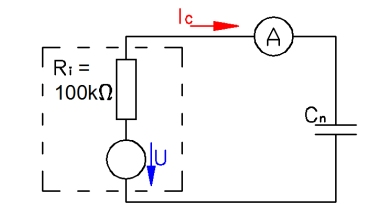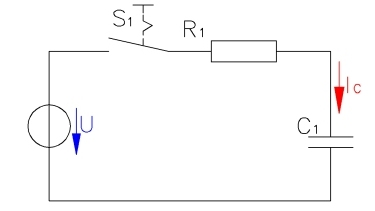Charging and discharching a capacitor will be examined in this lab. The capacitor stores energy in an electric field. Explore how a capacitor works.

saftey guidelines

Since the capacitor stores electric energy, the voltage across the capacitor can be high. Therefore a capacitor should be short-circuited before touching.

Pay attention to the plarity of the capcitor. Incorrect polarity will destroy the capacitor and result in a short circuit.

To avoid high currents during charging, a capacitor should always be operated in series with a current limitting resistance.# 1 Current through a capacitorChange the voltage and see charges build up on the capacitor plates.

C1 = 10 µF, C2 = 100 µF.

Note: Set the voltage, place the capacitor inside the circuit and immediately measure the maximum current.

1. Measure the current for a voltage of 0 to 12 V.

V in V IC1 in mA IC2 in mA 0 2 ...
1. Draw the characteristic V-IC1- and V-IC2-diagram.

2. Explain the relationships between voltage, charge, stored energy and capacitance.

# 2 Charging a capacitorYou will need a voltage source with a voltage V0 = 10 V and additionally a capacitor and resistor in series. Optionally the circuit can be closed with a switch S1.

1. Assemble the circuit with R = 100 kΩ and C = 100 µF.

2. Measure every 5 s the voltage VC and the current I, after closing the circuit with S1. Note: You can calculate the current with the voltage drop across the resistor R.

 t in s VC in V I in mA 0 5 10 ...
1. Draw the characteristic t-VC- und die t-I-diagram.

2. Repeat the measurement with different resistance and capacitance R = 22 kΩ and C = 100 µF as well as R = 22 kΩ and C = 47 µF. Choose an appropriate time interval.

3. Evaluate the relationships between charging time, capacitance and resistance.

# 3 Charging- and discharging a capacitorExplore how a capacitor works if switched on and off in a DC circuit.

Assemble the circuit. The frequency generator has an internal resistance of 50 Ω. Choose a time period of T = 1000 µs and an impulse time of ti = 500 µs ein. The voltage switches between 0  and 5 V.

The current is determined with the voltage drop across the resitor Rm = 100 Ω. The capacitors with capacitances of C1 = 10 nF, C2 = 100 nF, C3 = 1 µF, C4 = 10 µF, C5 = 100 µF will be used.

The duty cycle g is the rate between impulse time ti and cycle duration T. For ti = 500 µs and T = 1 ms the duty cycle is 50%. The capacitor will be charged and discharged every 500 µs.

1. Calculate the time constant τ15 and the resulting charging time. Plot the results in a table.

2. Measure the time-voltage-diagram with the oscilloscope across the capacitor and resistor. Plot the diagrams one below the other (2 cycle durations):
V = f(t), VC1 = f(t), VC2 = f(t), VC3 = f(t), VC4 = f(t) and VC5 = f(t).
V = f(t), IC1 = f(t), IC2 = f(t), IC3 = f(t), IC4 = f(t) and IC5 = f(t).

3. Calculate the voltage drop VC4 across and the current IC4 throug the capacitor after 2 µs.

4. Evaluate the time after 1τ, 2τ and 3τ in the diagram IC3 = f(t).

5. Optional: Calculate the maximum current through the capacitor.